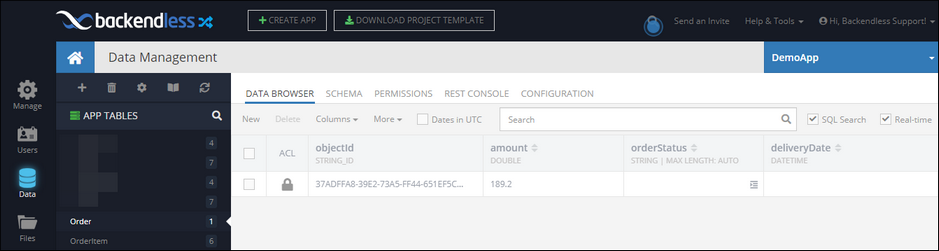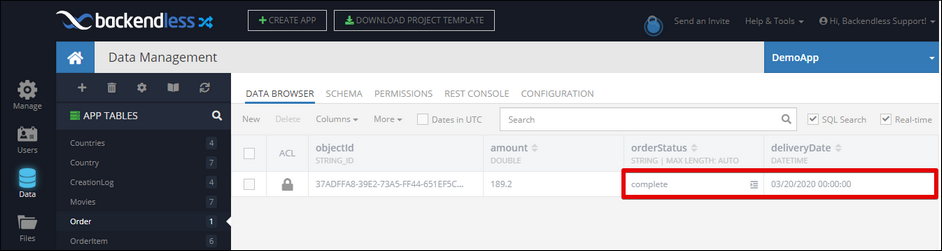# Updating a single object¶

Important

This operation updates a single object in the database. To update more than one object, use the bulkUpdate operation documented in the Updating multiple objects chapter of this guide.

To add this operation to a Unit Of Work instance, use the following methods:

The methods are organized by how the object to be updated is specified in the API. As you can see below, there are several ways to reference the object which will be updated in the database: explicit object reference, which is either using a `Dictionary` or a custom class or by using a result of a previous operation:

``````// Update the object represented by the "identifier" argument.
// The argument can be either a tableName, OpResult or OpResultValueReference object.
// The "changes" argument can be either a key/value map of changes or an object of your custom class.
// It must have a server assigned value for the objectId property.
unitOfWorkInstance.update<T>(T changes, [dynamic identifier ]);
``````

## Return Value¶

All methods listed above return an instance of the `OpResult` class which represents the updated object in the database. The `OpResult` object can be used as an argument in other operations in the same transaction (same `UnitOfWork` instance). For more information see the Operation Result chapter of this guide.

## Example¶

The examples below demonstrate the usage of the operation for all possible ways to reference the object that should be updated. This is the what the data table looks like before the transaction runs:``````final unitOfWork = UnitOfWork();

Map changes = {
"orderStatus": "complete",
};

unitOfWork.update(changes, "Order");

unitOfWork.execute().then((result) => print("transaction complete - \$result"));
``````

``````final unitOfWork = UnitOfWork();

final orderInstance = Order()
..orderStatus = "complete"

unitOfWork.update(orderInstance)

unitOfWork.execute().then((result) => print("transaction complete - \$result"));
``````
The Order class is defined as:
``````@reflector
class Order {
String orderStatus;
DateTime deliveryDate;
String objectId;
}
``````

``````final unitOfWork = UnitOfWork();

// compose query builder
final queryBuilder = DataQueryBuilder();

// set the query condition
queryBuilder.whereClause = "orderId = '031820-CV1'";

// we need only one object, this will help to speed it up
queryBuilder.pageSize = 1;

// add the find operation to the transaction
final findResult = unitOfWork.find("Order", queryBuilder);

// get a reference to the first object (order) from the result
final orderObjectRef = findResult.resolveTo(resultIndex: 0);

// compose a map of changes - notice the objectId is not needed there
Map changes ={
"orderStatus": "complete",